# Abstract

This paper presents an approach to a constitutive model for anisotropic quasi-brittle materials, developed in the framework of rate independent softening plasticity, involving a yield criterion in which an anisotropic friction tensor is involved. It turns out to be useful for materials characterized by ultimate behaviour which varies according to the direction, such as composite materials, anisotropic rocks, textiles, masonry. A geometrical representation of the limit domain in the case of plane stress, together with the results of laboratory tests is presented and discussed.

Anisotropy; failure criterion; friction; laboratory tests

# 1 INTRODUCTION

The intrinsic structure of a wide range of structural, geological and industrial materials, such as composites, masonry, wood, textiles and several types of rocks and clays, is the major cause of their anisotropic behaviour. The material response to the same stress state is strongly linked to the sampling orientation with respect to the principal stress axes. The position and the orientation of the clay particles, for example, define a sort of material geometry on which the stress geometry depends (Dialer, 1993Dialer, C., (1993). Masonry and rock mechanics - An interdisciplinary look at two related materials. Proceedings of the Sixth North American Masonry Conference, Philadelphia, Pennsylvania, U.S.A.). Similar considerations can be applied to a Representative Volume Element in order to describe the macroscopical behaviour of an equivalent anisotropic orientation of the fibers in a composite, the disposition of the bed and head joints in a masonry wall medium resulting from an homogenization technique (Aboudi, 1991Aboudi, J., (1991). Mechanics of composite materials - A unified micromechanical approach, Elsevier, Amsterdam-Oxford-New York-Tokyo.; Nemat Nasser, 1993Nemat Nasser, S., (1993). Micromechanics: overall properties of heterogeneous materials. Elsevier Science.). Moreover, anisotropic friction plays an important role in the formation of wrinkles and folds in textiles (Pabst et al., 2009Pabst, S., Thomaszewski, B., Straßer, W., (2009). Anisotropic Friction for Deformable Surfaces and Solids. Eurographics/ AC IGGRAPH Symposium on Computer Animation. E. Grinspun and J. Hodgins (Editors).). Some of the research efforts made in recent years to achieve a better understanding of constitutive behaviour of quasi-brittle materials have been developed in the framework of plasticity (Pietruszczak and Niu, 1992Pietruszczak, S., Niu, X., (1992). A mathematical description of macroscopic behaviour of brick masonry. International Journal of Solids and Structures 29(5): 531-546.). Constitutive models developed in this last field for concrete (Feenstra and De Borst, 1996Feenstra, P.H., De Borst, R., (1996). A composite plasticity model for concrete. International Journal of Solids and Structures 33(5): 707-730.; Wang et al., 2014Wang, Z. Jin, X.Y., Jin, N.G., Shah, A.A., Li, B., (2014). Damage based constitutive model for predicting the performance degradation of concrete. Latin American Journal of Solids and Structures 11(6): 907-924.) have been successfully applied to masonry structures. Unfortunately the postulates by (Drucker 1951Drucker, D.C., (1951). A more fundamental approach to stress-strain relations. Proc. First U.S. National Congress on Applied Mechanics.; 1988Drucker, D.C., (1988). Conventional and unconventional plastic response and representation. Applied Mechanics Review 41(4): 151-167.); Hill (1958)Hill, R., (1958). A general theory of uniqueness and stability in elasto-plastic solids. Journal of the Mechanics and Physics of Solids 6(3): 236-249.providing the framework for establishing the material stability have in recent times come under very strong challenge in relation to their validity for geomaterials. With this word are often termed the materials, such as soils, rocks, concrete and masonry, which exhibit frictional behaviour. The use of non-associated flow rules has been proposed as a way of accomodating such class of materials that, while experiencing dilation during shearing, nonetheless remain stable (Salençon, 1975Salençon, J., (1975). Applications of the theory of plasticity in soil mechanics. J. Wiley and Sons.; Famiyesin, 2001Famiyesin, O.O.R., (2001). Energy adaption of non-associated plasticity tangent matrices for symmetric solvers. Computer & Structures 79(13): 1233-1250.).

In computational plasticity the normality condition is assumed due to the numerical convenience and to the more developed theory (Simo and Taylor, 1985Simo, J.C., Taylor, R.L., (1985). Consistent tangent operators for rate-independent elastoplasticity. Computer Methods in Applied Mechanics and Engineering 48(1): 101-118.). Those who adopt the standard model argue about the inadmissibility of non-associated flow from thermodynamic point of view (Runesson and Sture, 1989Runesson, K., Sture, S, (1989). Stability of frictional materials. Journal of Engineering Mechanics ASCE 115(8): 1828-1833.).

Recently the theoretical and numerical problems about the variational formulation of non-associated law has been developed (Collins and Houlsby, 1997Collins, I.F., Houlsby, G.T., (1997). Application of thermomechanical principles to the modelling of geotechnical materials. Proceedings of the Royal Society A 453(1964): 1975-2001.), although the complexity of the problem welcomes more studies in the future.

Most contributions towards establishing a rational failure theory of quasi brittle materials exhibiting a strong anisotropic behaviour are phenomenological: experimental results are interpreted on the basis of a criterion which is assumed to be suitable for the observed mode of failure. Differences between formulae are mainly due to different failure hypotheses. Because of the difficulty of developing representative tests, both in number and in type, few attempts have been made to obtain a complete experimental failure domain (Zhou et al., 2012Zhou, Z., Wang, Z., Zhao, L., Shu, X., (2012). Experimental investigation on the yield behavior of Nomex honeycombs under combined shear-compression. Latin American Journal of Solids and Structures 9(4): 515-530.).

Theoretical approaches proposed in recent years involve elegant mathematical formulations (Pietruszczak et al., 1988Pietruszczak, S., Jiang, J., Mirza, F.A., (1988). An elastoplastic constitutive model for concrete. International Journal of Solids and Structures 24(7): 705-722.; Zmitrowicz, 2006Zmitrowicz, A., (2006). Models of kinematics dependent anisotropic and heterogeneous friction. International Journal of Solids and Structures 43(14-15): 4407-4451.), but often need sophisticated laboratory tests to determine material parameters for the identification of the failure domain (Babu et al., 2006Babu, R.R., Benipal, G.S., Singh, A.K., (2006). Plasticity-based constitutive model for concrete in stress space. Latin American Journal of Solids and Structures 3(4): 417-441.).

A thorough discussion on failure criteria, together with an extended list of references, can be found in the papers by Duveau et al. (1998)Duveau, G., Shao, J.F., Henry, J.P., (1998). Assessment of some failure criteria for strongly anisotropic geomaterials. Mechanics of Cohesive-Frictional Materials 3(1): 1-26. for geotechnical materials, in the paper by Anthoine (1992Anthoine, A., (1992). Derivation of the in-plane elastic characteristics of masonry through homogenization theory. Int. Journal of Solids and Structures 32(2): 137-163.) for masonry and in the paper by Zmitrowicz (2006)Zmitrowicz, A., (2006). Models of kinematics dependent anisotropic and heterogeneous friction. International Journal of Solids and Structures 43(14-15): 4407-4451. for a large class of materials (composites, cork, crystals, wood, polymers, ceramics and layer-lattice materials).

The theory proposed in this paper, whose general framework is outlined in section 2, provides a failure criterion for quasi brittle materials in which the existence of an anisotropic friction tensor is postulated (Frunzio et al., 2000Frunzio, G., Gesualdo, A., Monaco M., (2000). Failure behaviour of brick masonry. Masonry International 14(2): 41-45.).

The yield condition, based on the Mohr-Coulomb relation, involves six material parameters. For the identification of the domain thus determined the only classical shear box test is needed, since the principal friction coefficients can be obtained by performing the same test according to the three principal friction directions, if those are easily known by examining the material structure.

A geometrical representation of the failure domain is provided in section 3 in the case of plane stress. It is shown that the agreement between the theory and experiments is reasonably good, although a direct comparison cannot be performed since the friction data are not available in literature. Experimental tests arranged for the identification of the criterion and performed on masonry samples are considered and discussed in this paper.

# 2 THE CONSTITUTIVE MODEL

Reference is made to an anisotropic inelastic body with softening constitutive law, defined over a domain Ω on which loads t0 and displacement u 0 are assigned respectively on the parts ∂Ωu and ∂Ωt of the boundary ∂Ω, while body forces b 0 are assigned in Ω as in Figure 1. The governing relations are developed with the assumptions that strains and displacements are small.

The static and kinematic fields are referred to a Cartesian co-ordinate system:

With the above assumptions, the compatibility conditions for the body Ω give: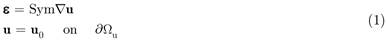where ε represents the small strain tensor. The equilibrium conditions for the body Ω give:where σ represents the Cauchy stress tensor and n is the outward normal. The constitutive problem for the body Ω is governed by the following relations:where ω is the strain energy density function corresponding to an elastic behaviour for the body Ω. If a linear elastic behaviour is assumed, ω is a quadratic function of ε; the displacement u is considered as the sum of an elastic part ue and an inelastic part ui. The behaviour of the body is defined in two conjugate spaces of generalized variables: the set of internal kinematic variables α and the conjugate set of statical internal variables s define the inelastic behaviour, while the elastic behaviour is defined by the kinematic variables ue and static variables σ. The plastic multipliers are expressed by λ.

The tractions t and the static internal variables α are given as derivatives of a free energy function density ψ(ue, α), non convex to reproduce softening. The yield function f(σ, s) is a different function from the plastic potential g(σ, s). This corresponds to the assumption of nonassociated plasticity, that is a generalized nonstandard material. The problem to be solved is to find the equilibrium configurations of the body Ω under the assigned loads.

# 3 THE YIELD FUNCTION

As yield function can be considered that one proposed by the authors for anisotropic frictional materials (Frunzio et al., 2000Frunzio, G., Gesualdo, A., Monaco M., (2000). Failure behaviour of brick masonry. Masonry International 14(2): 41-45.). The criterion considers a quasi-brittle material in which the admissible stress states are those given by the classical Mohr-Coulomb relation: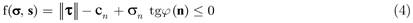in which, if Πn is a plane with normal n (Figure 2):

• • τ is the total shear stress on the plane Πn and the symbol ||•|| is the Euclidean norm;

• • cn is the material cohesion relative to the plane with normal n given by: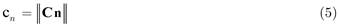• • σn is the normal stress on Πn;

• • tgφ(n) is the friction coefficient on Πn. It is supposed varying according to the following law: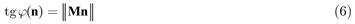In (5) and (6) the existence of a cohesion tensor C and a friction tensor M has been postulated. As shown in the following, three principal friction axes, coinciding with the principal orthotropic axes, can be recognized in an orthotropic material such as brick masonry. With reference to the principal friction frame the tensors C and M can be expressed as:where:

• • μi = tgφi is the friction coefficient [i = 1, 2, 3];

• • φi is the friction angle [i = 1, 2, 3];

• • ci is the material cohesion [i= 1, 2, 3].

In order to obtain the relation (4) in the principal friction frame, the stress tensor T is given by:

T = QTSQ

with S diagonal stress tensor in the principal stress frame and Q orthogonal tensor governing the reference frame change, from the principal friction frame to the principal stress one. The limit condition in (4) becomes:The set K of admissible stress states according to (4) is convex (Piccolroaz and Bigoni, 2009Piccolroaz, A., Bigoni, D., (2009). Yield criteria for quasibrittle and frictional materials: A generalization to surfaces with corners. International Journal of Solids and Structures 46(20): 3587-3596.), since every convex combination T3 of two admissible stress states T1 and T2 is an element of K:The set K is convex if and only if every convex combination T3 of two admissible stress states T1 and T2 is an element of K.

So that: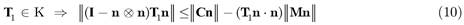multiplying (10) and (11) respectively for λ and (1 - λ) the above relations can be written: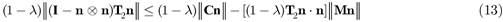summing (12) and (13), the following inequality can be written:from the triangle inequality of normed vector spaces and the linearity of operators T1 and T2 follows:while the second member of (14) can be written as: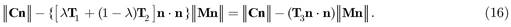By (14), (15) and (16) finally one has:and so the set K is convex.

Differently from the classical Mohr-Coulomb yield condition, in which an isotropic friction tensor is involved, a geometrical representation of the proposed limit domain in the principal stress space is somewhat difficult. It must be noted that the problem of convexity of the yield domain is a fundamental question. In the cases of non standard materials, where the convexity cannot be derived from the postulate by Drucker, a non convex domain requires appropriate loading paths for the solution of the problem while the convexity assures computational advantages (Lemaitre and Chaboche, 1990Lemaitre, J, Chaboche, J.L., (1990) Mechanics of solid materials. Cambridge University Press, Cambridge, U.K.; Maugin, 1992Maugin, G.A., (1992). The thermomechanics of plasticity and fracture. Cambridge University Press, Cambridge, U. K.).

The proposed yield criterion can be applied also to assess the failure under combined loads for more complex engineering problem as shown in (Fraldi et al., 2012), the local collapse of the cavities in anisotropic rock banks in the sense of the methodology presented in (Nunziante et al., 2001Nunziante, L., Gesualdo, A., Minutolo, V., (2001). On the local collapse in soft rock bank cavities. Journal of Geotechnical and Geoenvironmental Engineering ASCE 127(12): 1037-1042.) and also with a finite element validation (Gesualdo et al., 2001Gesualdo, A., Nunziante, L., Minutolo, V., (2001). A kind of failure in Mohr-Coulomb soil cavities. Canadian Geotechnical Journal 38(6): 1314-1320.).

As plastic potential can be considered a function formally similar to that proposed for the yield criterion, where the friction tensor is replaced by a "dilatancy tensor" (Salencon, 1975Salençon, J., (1975). Applications of the theory of plasticity in soil mechanics. J. Wiley and Sons.), of the form:where the dilatancy tensor is given by: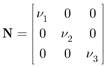A numerical solution of the problem is possible, so that a weak formulation allowing the spatial discretization through finite elements, is proposed. Assuming that the compatibility and the constitutive laws are locally fulfilled on the body Ω, a weak formulation involves the relations (19) and (20). The equilibrium conditions can be expressed by the classical formulation of the virtual work:where:

U = {u : u regular in Ω, u = u0 on ∂Ωu}

U0 = {∂u : ∂u regular in Ω, ∂u = 0 on ∂Ωu}

While the constitutive equations are given by:where:

T = {∂σ = σ* - σ, ∂s = s* - s : f(σ*, s*) ≤ 0}

The above relations can be obtained solving an extremum problem for the functional: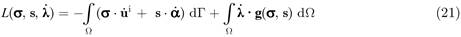The sub-stationariness condition of the functional L(σ, s,) gives in fact a point (σ, s,) that satisfies the integral relations in (20). The formulation can be the basis for space discretization by means of finite elements, replacing the field variables σ, u, s and α, conjugate in pairs, with the interpolations of the corresponding nodal quantities (Bolzon and Corigliano, 1997Bolzon, G., Corigliano, A., (1997). A discrete formulation for elastic solids with damaging interfaces. Computer Methods in Applied Mechanics and Engineering 140(3-4): 329-359.).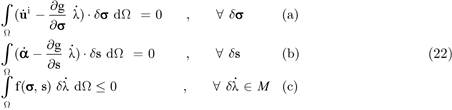where:# 4 MASONRY AS ANISOTROPIC QUASI-BRITTLE MATERIAL: EXPERIMENTS AND COMPARISONS

Brick masonry can be considered as an anisotropic material in which the principal material directions are those of the bed and head joints (Figure 3), and the above considerations can be applied. To determine the failure criterion for masonry, laboratory tests have been performed in order to evaluate the yield states for brick masonry and match the results with literature yield domains.

Tests arranged on masonry cubes have been performed for the determination of the mechanical parameters above defined. Brick masonry cubes, 15 × 15 × 15 cm have been tested by means of a shear box. Currently produced clay bricks and a pozzolanic lime mortar have been employed to build up the cubes, whose texture is represented in Figure 4 (a).

Because of the particular geometry of the specimen, the three principal friction axes have been assumed coinciding with the three orthogonal axes of the cube.

After one year curing the shear tests have been performed according the three axes of the specimen and with different values of the vertical pressure. The vertical load V, constant during the test, and the variable horizontal load H have been measured by means of a load cell, while horizontal and vertical displacements have been evaluated through inductive displacement transducers.

The scheme of the Casagrande box is reported in Figure 4 (b), while a photograph of a specimen after the test is shown in Figure 5. The results of the experimental tests are reported in the following:

Figure 6:
Horizontal load displacement curve (left) and evaluation of material parameters (right) for the masonry specimen according to direction 1.

Figure 7:
Horizontal load displacement curve (left) and evaluation of material parameters (right) for the masonry specimen according to direction 2.

Figure 8:
Horizontal load displacement curve (left) and evaluation of material parameters (right) for the masonry specimen according to direction 3.

As expected, since the texture of the brick masonry, the specimen has shown different behaviour according the three test directions. The three values of the friction angle, both with the values of the material parameter cn , are reported in the following table (Frunzio et al., 1999).In this case the dilatancy can be obtained as a function of the geometrical parameters (Stupkiewicz and Mróz, 2001Stupkiewicz, S., Mróz, Z., (2001). Modelling of friction and dilatancy effects at brittle interfaces for monotonic and cyclic loading. Journal of Theoretical and Applied Mechanics 3(39): 707-739.):

where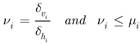A geometrical representation of the failure surface is possible in the case of plane stress. Let σ1 and σ2 be the principal non zero stresses, laying in the principal friction plane μ1, μ2, the failure domain can be obtained in the space (σ1, σ2, ϑ), where ϑ is the angle between the principal friction frame and the principal stress one.

In this case the tensor Q becomes:while the stress tensor is given by:The limit condition (8) provides, as n changes, a set of domains whose boundaries are conic sections. The intersection gives, for every ϑ, the limit domain, represented and discussed in the following pictures, that is the set of admissible stress states: it is convex as it can be expected for brittle materials (Lin and Bazant, 1986Lin, F.B., Bazant, Z.P., (1986). Convexity of smooth yield surface of frictional materials. Journal of Engineering Mechanics Division ASCE 112(11): 1259-1262.; Jiang and Pietruszczak, 1988Jiang, J., Pietruszczak, S., (1988). Convexity of yield loci for pressure sensitive materials. Computers & Geotecnics 5(1): 51-63.).

The general statement of the criterion (8) is useful to numerically determine the safe stress states, though an exact solution of the minimum problem associated with the determination of the envelope boundary involves a complicated non linear equation system. As a result, the following pictures are obtained drawing the set of curves corresponding to discrete values of n (Wolfram, 2003Wolfram, S., (2003). The Mathematica Book, Fifth Edition. Wolfram Media ISBN 1-57955-022-3.). Figure 10 shows five failure surface sections in the plane σ1 - σ2, corresponding to five different values of the angle ϑ.

Figure 10:
Failure surface sections for different values of ϑ with material parameters given in table 1 (experimental data).

The shape of the domain is similar to the experimental ones evaluated by (Page 1981Page, A.W., (1981). The biaxial compressive strength of brick masonry. Proceedings of Institution of Civil Engineers 71(3): 893-906. and 1982Page, A.W., (1982). An experimental investigation of the biaxial strength of brick masonry. Proceedings of the 6th International Brick Conference, Rome, Italy.) on brick masonry panels (Figure 11), and by several authors on concrete (Figure 12).

Figure 11:
Experimental results for brick masonry panels subjected to biaxial stress in (σ1, σ2) plane.

Figure 12:
Experimental results for low strength concrete (a) and high strength concrete (b) subjected to biaxial stress.

In particular, in Figure 12 (a) are reported the results of biaxial tests performed on two different types of low strength concrete by Kupfer et al. (1969)Kupfer, H.B., Hilsdorf, H.K., Rush, H., (1969). Behaviour of concrete under biaxial stresses. ACI Journal 66(8): 656-666., while in Figure 12 (b) are reported the results of biaxial tests performed on two similar types of high strength concrete by the same authors (squares) and by Andenaes et al. (1977)Andenaes, E., Gerstle K., Ko, H.Y., (1977). Response of mortar and concrete to biaxial compression. Journal of the Engineering Mechanics Division ASCE 103(4): 515-525 (triangles).

The similarity is strongly evident not only in the biaxial compression range, but in the biaxial tension and in the tension-compression range, in which the experimental test performing is somewhat difficult.

A direct numerical comparison cannot be performed, since in literature the friction data are not available. Nevertheless, a tentative drawing of the proposed domain is shown dashed in both the pictures. The values of the material parameters have been evaluated a posteriori and reported in Table 2:

Table 2:
Values of the material parameters for the literature tests.

Both brick masonry and concrete present transversal isotropic friction properties (Proença and Pituba, 2003Proença, S.P.B., Pituba, J.J.C., (2003). A damage constitutive model accounting for induced anisotropy and bimodular elastic response. Latin American Journal of Solids and Structures 1(1): 101-117.). The limit domain is reported in Figs. 13-15 for ϑ = 0 to examine the influence of the different material parameters.

Figure 13:
Variation of the yield domain according the variation of μ1 with μ2 = 1.0, μ3 = 1.2, c = 0.2 N/mm2, ϑ = 0º.

Figure 14
Variation of the yield domain according the variation of μ3 with μ1 = 0.8, μ2 = 1.0, c = 0.2 N/mm2, ϑ = 0º.

Figure 15
Influence of c on the variation of the yield domain with μ1 = 0.8, μ2 = 1.0, μ3 = 1.7, ϑ = 0º.

In the Figs 13 and 14, the influence of the friction coefficient μ1 and μ3, respectively, can be observed. The domain is in general non-symmetric, with respect to the line bisecting I and IV quadrant. In the Figure 13 the progressive symmetrization of the domain without change of shape, as the values of μ1 approaches the value of μ2, is shown.

The perfect symmetry represents an ultimate transversely isotropic behaviour, with symmetry axis orthogonal to the stress plane, and μ1 = μ2.

In Figure 14 it can be noted that the domain size, without changing of shape, is strongly increasing with the increase of μ3, which is the friction coefficient on the stress plane.

A similar increase of the limit domain can be observed in Figure 15, where the influence of the parameter c is shown.

It appears, by the analysis of the above pictures, that the effect of both the friction coefficient μ3 and the parameter c on the failure domain extent is greater than the influence of the friction coefficients μ1 and μ2: the out-of-plane material characteristics are taken into account and play an important role in the material failure.

# 5 CONCLUSIONS

A failure criterion with a relatively simple analytical structure has been presented to model the ultimate behaviour of anisotropic quasi-brittle materials in which a variation of the friction coefficient according the direction can be recognized (composite materials, anisotropic rocks, textiles, masonry). The criterion is based on the classical Mohr-Coulomb limit condition in which a friction tensor is involved.

The criterion has easy application, due both to the simple formulation and to the simple laboratory tests involved, since the only test required for the material identification is the classical shear box test to determine the friction and the cohesion tensors.

The only hypothesis of three different material friction angles gives as a result a limit convex domain spreading largely in the compression-compression range, and with limited area in the tension range: this represents typically the behaviour of quasi-brittle materials with limited tensile strength.

In the case of plane stress state presented above, the influence of the transversal friction coefficient is remarked: the dimensions of the limit domain proposed are strongly influenced by that material parameter.

Though a detailed analysis of the limit domain in plane stress state has been presented, the general statement of the criterion is also useful in the analysis of three-dimensional stress state.

An application to the constitutive behaviour of masonry has been presented. The framework of plasticity with internal variables provides a coherent description of the softening problem to represent the behaviour of masonry.

# References

• Aboudi, J., (1991). Mechanics of composite materials - A unified micromechanical approach, Elsevier, Amsterdam-Oxford-New York-Tokyo.
• Andenaes, E., Gerstle K., Ko, H.Y., (1977). Response of mortar and concrete to biaxial compression. Journal of the Engineering Mechanics Division ASCE 103(4): 515-525
• Anthoine, A., (1992). Derivation of the in-plane elastic characteristics of masonry through homogenization theory. Int. Journal of Solids and Structures 32(2): 137-163.
• Babu, R.R., Benipal, G.S., Singh, A.K., (2006). Plasticity-based constitutive model for concrete in stress space. Latin American Journal of Solids and Structures 3(4): 417-441.
• Bolzon, G., Corigliano, A., (1997). A discrete formulation for elastic solids with damaging interfaces. Computer Methods in Applied Mechanics and Engineering 140(3-4): 329-359.
• Collins, I.F., Houlsby, G.T., (1997). Application of thermomechanical principles to the modelling of geotechnical materials. Proceedings of the Royal Society A 453(1964): 1975-2001.
• Dialer, C., (1993). Masonry and rock mechanics - An interdisciplinary look at two related materials. Proceedings of the Sixth North American Masonry Conference, Philadelphia, Pennsylvania, U.S.A.
• Drucker, D.C., (1951). A more fundamental approach to stress-strain relations. Proc. First U.S. National Congress on Applied Mechanics.
• Drucker, D.C., (1988). Conventional and unconventional plastic response and representation. Applied Mechanics Review 41(4): 151-167.
• Duveau, G., Shao, J.F., Henry, J.P., (1998). Assessment of some failure criteria for strongly anisotropic geomaterials. Mechanics of Cohesive-Frictional Materials 3(1): 1-26.
• Famiyesin, O.O.R., (2001). Energy adaption of non-associated plasticity tangent matrices for symmetric solvers. Computer & Structures 79(13): 1233-1250.
• Feenstra, P.H., De Borst, R., (1996). A composite plasticity model for concrete. International Journal of Solids and Structures 33(5): 707-730.
• Fraldi, M., Nunziante, L., Gesualdo, A., Guarracino, F., (2010). On the bounding of multipliers for combined loading. Proceedings of the Royal Society A 466(2114): 493-514.
• Frunzio, G., Gesualdo, A., Monaco M., (2000). Failure behaviour of brick masonry. Masonry International 14(2): 41-45.
• Gesualdo, A., Nunziante, L., Minutolo, V., (2001). A kind of failure in Mohr-Coulomb soil cavities. Canadian Geotechnical Journal 38(6): 1314-1320.
• Hill, R., (1958). A general theory of uniqueness and stability in elasto-plastic solids. Journal of the Mechanics and Physics of Solids 6(3): 236-249.
• Jiang, J., Pietruszczak, S., (1988). Convexity of yield loci for pressure sensitive materials. Computers & Geotecnics 5(1): 51-63.
• Kupfer, H.B., Hilsdorf, H.K., Rush, H., (1969). Behaviour of concrete under biaxial stresses. ACI Journal 66(8): 656-666.
• Lemaitre, J, Chaboche, J.L., (1990) Mechanics of solid materials. Cambridge University Press, Cambridge, U.K.
• Lin, F.B., Bazant, Z.P., (1986). Convexity of smooth yield surface of frictional materials. Journal of Engineering Mechanics Division ASCE 112(11): 1259-1262.
• Maugin, G.A., (1992). The thermomechanics of plasticity and fracture. Cambridge University Press, Cambridge, U. K.
• Nemat Nasser, S., (1993). Micromechanics: overall properties of heterogeneous materials. Elsevier Science.
• Nunziante, L., Gesualdo, A., Minutolo, V., (2001). On the local collapse in soft rock bank cavities. Journal of Geotechnical and Geoenvironmental Engineering ASCE 127(12): 1037-1042.
• Pabst, S., Thomaszewski, B., Straßer, W., (2009). Anisotropic Friction for Deformable Surfaces and Solids. Eurographics/ AC IGGRAPH Symposium on Computer Animation. E. Grinspun and J. Hodgins (Editors).
• Page, A.W., (1981). The biaxial compressive strength of brick masonry. Proceedings of Institution of Civil Engineers 71(3): 893-906.
• Page, A.W., (1982). An experimental investigation of the biaxial strength of brick masonry. Proceedings of the 6th International Brick Conference, Rome, Italy.
• Piccolroaz, A., Bigoni, D., (2009). Yield criteria for quasibrittle and frictional materials: A generalization to surfaces with corners. International Journal of Solids and Structures 46(20): 3587-3596.
• Pietruszczak, S., Jiang, J., Mirza, F.A., (1988). An elastoplastic constitutive model for concrete. International Journal of Solids and Structures 24(7): 705-722.
• Pietruszczak, S., Niu, X., (1992). A mathematical description of macroscopic behaviour of brick masonry. International Journal of Solids and Structures 29(5): 531-546.
• Proença, S.P.B., Pituba, J.J.C., (2003). A damage constitutive model accounting for induced anisotropy and bimodular elastic response. Latin American Journal of Solids and Structures 1(1): 101-117.
• Runesson, K., Sture, S, (1989). Stability of frictional materials. Journal of Engineering Mechanics ASCE 115(8): 1828-1833.
• Salençon, J., (1975). Applications of the theory of plasticity in soil mechanics. J. Wiley and Sons.
• Simo, J.C., Taylor, R.L., (1985). Consistent tangent operators for rate-independent elastoplasticity. Computer Methods in Applied Mechanics and Engineering 48(1): 101-118.
• Stupkiewicz, S., Mróz, Z., (2001). Modelling of friction and dilatancy effects at brittle interfaces for monotonic and cyclic loading. Journal of Theoretical and Applied Mechanics 3(39): 707-739.
• Wang, Z. Jin, X.Y., Jin, N.G., Shah, A.A., Li, B., (2014). Damage based constitutive model for predicting the performance degradation of concrete. Latin American Journal of Solids and Structures 11(6): 907-924.
• Wolfram, S., (2003). The Mathematica Book, Fifth Edition. Wolfram Media ISBN 1-57955-022-3.
• Zhou, Z., Wang, Z., Zhao, L., Shu, X., (2012). Experimental investigation on the yield behavior of Nomex honeycombs under combined shear-compression. Latin American Journal of Solids and Structures 9(4): 515-530.
• Zmitrowicz, A., (2006). Models of kinematics dependent anisotropic and heterogeneous friction. International Journal of Solids and Structures 43(14-15): 4407-4451.

# Publication Dates

• Publication in this collection
Aug 2015